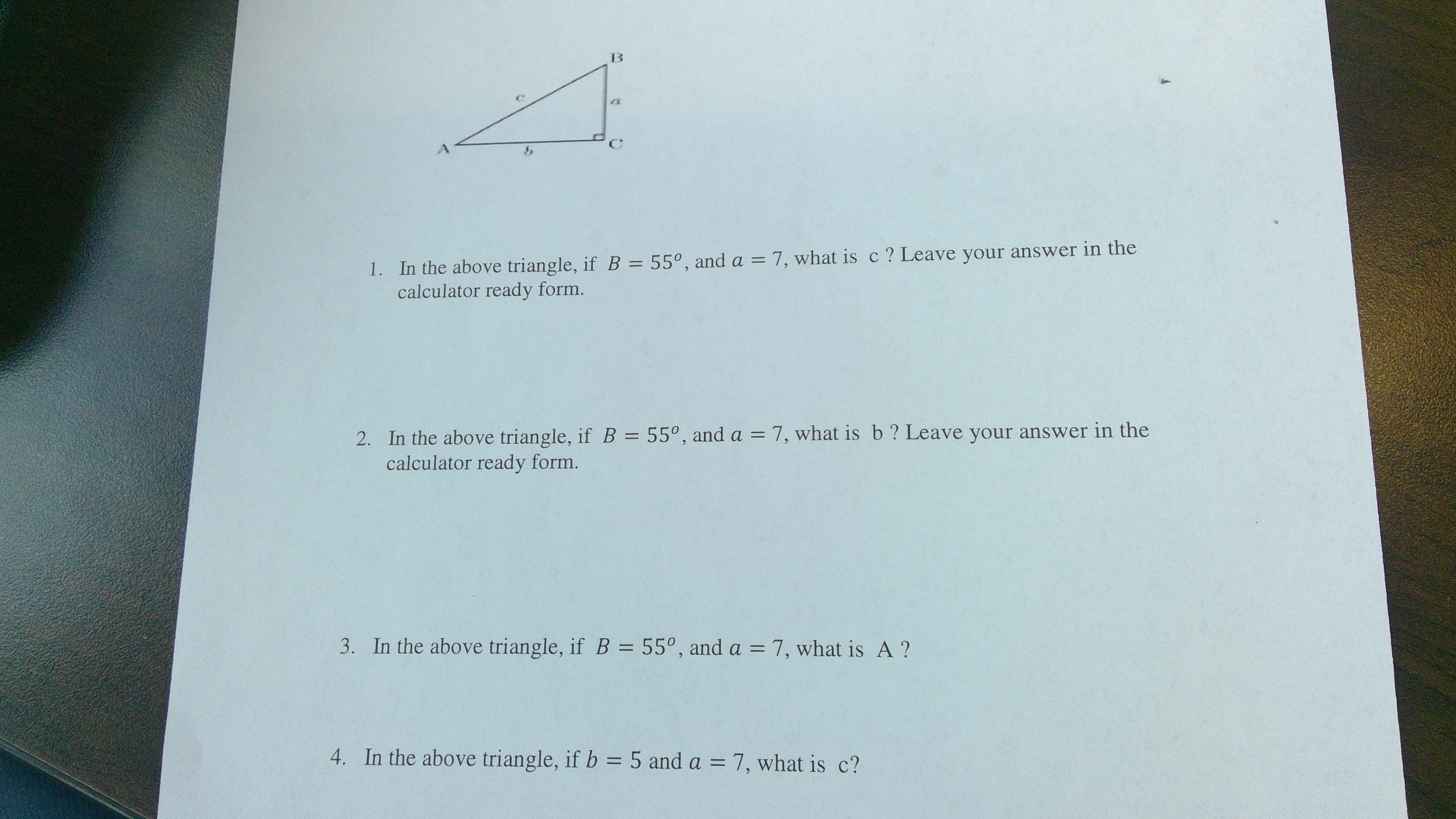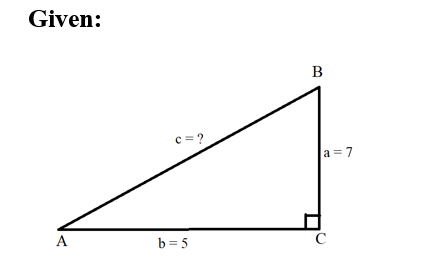# 1. In the above triangle, ifB = 55°, and a = 7, what is c ? Leave your answer in thecalculator ready form.2. In the above triangle, if B = 55°, and a = 7, what is b ? Leave your answer in thecalculator ready form.3. In the above triangle, if B = 55°, and a = 7, what is A ?%3D4. In the above triangle, if b = 5 and a = 7, what is c?%3D

Question
9 views

How do you solve question 4 and can you show me step by step?help_outlineImage Transcriptionclose1. In the above triangle, ifB = 55°, and a = 7, what is c ? Leave your answer in the calculator ready form. 2. In the above triangle, if B = 55°, and a = 7, what is b ? Leave your answer in the calculator ready form. 3. In the above triangle, if B = 55°, and a = 7, what is A ? %3D 4. In the above triangle, if b = 5 and a = 7, what is c? %3D fullscreen
check_circle

Step 1...

### Want to see the full answer?

See Solution

#### Want to see this answer and more?

Solutions are written by subject experts who are available 24/7. Questions are typically answered within 1 hour.*

See Solution
*Response times may vary by subject and question.
Tagged in

### Trigonometry